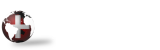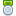####Author Topic: JSON DIM LOAD  (Read 471 times)

#### bteixeira

• Silver Member
•• Posts: 26« on: September 15, 2021, 09:44:24 AM »
If I have a JSON array that's basically different records something like:

[{"name":"Alice","type":"A","balance":"123.45"},{"name":"Bob","type":"A","balance":"6543.21","extra-field":"1","extra2":"2"}]

if I do

The results are:
array\$["1.name"]="Alice"
array\$["1.type"]="A"
array\$["1.balance"]="123.45"
array\$["2.name"]="Bob"
array\$["2.type"]="A"
array\$["2.balance"]="6543.21"
array\$["2.extra-field"]="1"
array\$["2.extra2"]="2"

What I'm looking for is a way to get the JSON string of the whole first element.  So something like
array\$["1"]="{""name"":""Alice"",""type"":""A"",""balance"":""123.45""}"

Is there a way to do that?

#### Mike King« Reply #1 on: September 15, 2021, 10:28:42 AM »
There is no "Magic" way to extract the first element only.

My suggestion would be to copy the elements whose name start with "1." to another array as in:

json\$="[{'name':'Alice','type':'A','balance':'123.45'},{'name':'Bob','type':'A','balance':'6543.21','extra-field':'1','extra2':'2'}]"
dim one\$
for k\$ index array\${all}
if mid(k\$,1,2)="1." \
then one\$[k\$(3)]=array\$[k\$]
next k\$
json1\$=dim(list one\${all})
print json1\$

->run
{"name":"Alice","type":"A","balance":"123.45"}